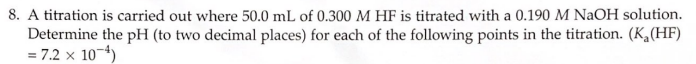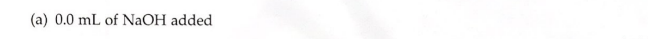# 8. A titration is carried out where 50.0 mL of 0.300 M HF is titrated with a 0.190 M NaOH solution. Determine the pH (to two decimal places) for each of the following points in the titration. ( Ka(HF) = 7.2 x 10^-4) (a) 0.0 mL of NaOH added